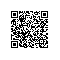# T-SQL中多行数据合并到一行(note)

id value
—————
1 aa
1 bb
2 aaa
2 bbb
2 ccc

SELECT id,
[val]=( SELECT [value] +','
FROM tb AS b
WHERE b.id = a.id
FOR XML PATH('') )
FROM tb AS a

1 aa,bb,
1 aa,bb,
2 aaa,bbb,ccc,
2 aaa,bbb,ccc,
2 aaa,bbb,ccc,

SELECT id,
[val]=( SELECT [value] +','
FROM tb AS b
WHERE b.id = a.id
FOR XML PATH('') )
FROM tb AS a
GROUP BY id

1 aa,bb,
2 aaa,bbb,ccc,

SELECT id,
[val]=STUFF( (SELECT ','+[value]
FROM tb AS b
WHERE b.id = a.id
FOR XML PATH('')) , 1 , 1 , '' )
FROM tb AS a
GROUP BY id

1 aa,bb
2 aaa,bbb,ccc

STUFF 函数将字符串插入另一字符串。它在第一个字符串中从开始位置删除指定长度的字符；然后将第二个字符串插入第一个字符串的开始位置。
STUFF ( character_expression , start , length ,character_expression_insert )

SELECT id,
[val]= REPLACE( (SELECT [value] AS [data()]
FROM tb AS b
WHERE b.id = a.id
FOR XML PATH('')) , ' ', ',')
FROM tb AS a
GROUP BY id使用钉钉扫一扫加入圈子
+ 订阅